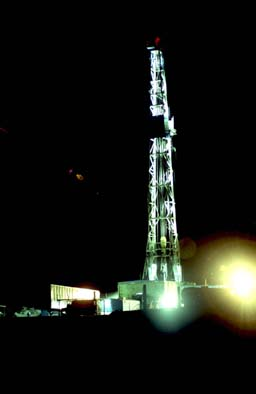## Objective Analysis

Kevin T. Kilty

### Introduction

There are two situations in which an automatic interpretation of geophysical data is useful. Sometimes our clients agree only to a geophysical budget that is inadequate for both collecting and interpreting data. Perhaps 80% of the available budget is consumed in planning and data collection, leaving us to shoe-horn interpretation into what remains. We can provide costly forward or inverse modeling only selectively, and if the client has little or no geophysical staff no better interpretation is ever available. Even when a survey is well planned and executed, and there is enough independent information from physical measurements and drill-holes to produce a very accurate interpretation, geophysical results may languish in obscurity for years. An objective analysis provides some way of examining all the data within an available budget. Another instance is a project involving a large amount of routine and unsophisticated analysis. For example, searching a large data set for a particular anomaly, or using magnetic or conductivity measurements to map lithology.

ln this paper we summarize characteristics of a few objectlve methods with which we are familiar. We do not intend to make a comprehensive review of inverse methods. ln particular, we will not discuss non-linear iterative methods. Most of these work from equations linearized in the neighborhood of a preferred solution. They require too much user input to consider them automatic.

### Problems in Objective Analysis

An ideal analyzer would accept reduced geophysical data, perhaps some independent information also, and return an objective interpretation. Building such an analyzer involves substantial, but interesting, operational problems.

First, there is the problem of uniqueness of the resulting analysis. Geophysical problems rarely admit a unique inverse, and ad hoc assumptions constraining a general inverse often lead to peculiar solutions. To restrict solutions to a reasonable range an analyzer must implement a model appropriate to a specific problem, or at least a model robust in its parameters. Some parameters may not be estimable at all, and others only as functions of one another.

Second, terrain and cultural effects influence the resulting interpretation unless data processing somehow eliminates them. Acceptable methods of correcting for terrain are available for gravity and VLF dip-angle (Karous, 1979). However, these do not eliminate terrain effects entirely because the observations are not continued to a level plane. After correcting observations for sources between the point of observation and datum, an analyzer could interpolate the observed data onto a uniform grid and then continue them, analytically, to a uniform level (Aronov, l970). Cultural effects are a separate consideration. Generally they have such short wavelengths that despiking and measurement response smoothing (Hayden, l987) eliminates them effectively. However, sampling or subsequent data conditioning might alias the spectra of such effects. A process as simple as interpolatlng irregular observations onto a uniform grid may increase lnterference between noise and signal, unless a person does it in a way to avoid aliasing.

Third, obtaining an accurate estimate of spectrum involves three considerations; avoiding aliasing of the raw data or its resampling, windowing of the data to improve frequency resolution, and conditioning the data in advance to reduce noise.

Finally, there is presentation of the results. Naturally one tends to present results as a cross section. This is not always possible or desirable. With potential fields data a cross section is not unique and may reflect more about characteristics of the analysis method than it does about the geophysical data. We illustrate abstract cross sections using correlation coefficient and F statistic and profiles of source strength. Others types of presentations may be useful in specific problems.

### Linear Filters

Most uses of linear filtering, exclusive of seismic geophysics, are for transforming potential fields--analytic continuation, reduction-to-pole, and so forth. However, linear filtering is also an objective analyzer. VLF dip-angle data, for example, are difficult to interpret in raw form because zero-crossings constitute the signal. Zero-crossings are not easy to identify, especially when they are superimposed on a trend. ln addition, there is the confusing influence of noise and uncertainty about which zero-crossings are slgnificant and which are false. Frazer (l969) designed a linear filter to transform proper zero-crossings into positive peaks, and the false crossings into negative troughs. ln addition his filter rejects low-frequency trends and high-frequency noise.

Frazer filtering is a prototype objective analyzer that transforms a measured potential field into its causative sources. lt is not very accurate, but a filter need not be very accurate to be useful. ln fact a highly accurate filter is of no use if its results are presented inappropriately. For instance, Karous and Hjelt (l983) designed an accurate dip-angle filter which accepts equally spaced readings and returns an estimated source current at a depth equal to the sample spacing. By repeating this process with data resampled at successively greater intervals, a person produces a psuedo-section of current distribution, as in Figure 1.Figure 1. Output of a VLF filter.

This unfortunate presentation suggests a geological cross section. However, no inverse method produces a unique cross section from a potential field without independent information on the depth of the source, and the cross section itself is therefore misleading. The behavior of Karous' and Hjelt's dip- angle filter applies to linear filters of other potential fields and also to generalized inversions (Shuey and Wannamaker, l978). VLP data are smoothed prior to each resampling to avoid aliasing and to stabilize the filter. The deeper current distribution, therefore, satisfies a smoothed version of the observed field. This forces long wavelength components of the source to greater depth whether or not they actually belong there. Continuity of sources and any apparent dip on such cross sections are not necessarily real, but principally a characteristic of the method.

The original dip-angle data of Figure 1 were taken across a fence and processed using the 8 point filter of Karous and Hjelt. The sample spacing is too wide, and the raw data are therefore aliased. Thus, the reconstructed current distribution is smooth despite being far below its true location. ln addition to suggesting vertical continuity, Figure 1 shows an apparent dip to the left from a systematic bias in the resampling.

Linear filtering to recover source distributions is also applicable to magnetic or gravity data. For example, assuming relief on a surface to be small compared to its depth (z), gravity observations are transformed to relief by the filter.

R=exp((kx+ky)z)/2PI

The equivalent filter for magnetic data is P*R where P is the pseudo-gravity operator--that is, the Fourier transform of Poisson's relation. The relief filter, whether applied to gravity or magnetic data, produces different relief for each assumed value of depth. A unique result is possible only when depth is specified by independent means, such as a borehole log.

ln Figure 2 rock units of varying susceptibility abut one another and are covered wlth a uniform thickness of alluvium. Suppose we wish to map rock type using magnetic measurements. The map is easier to produce if we transform magnetic measurements into a distribution of rock magnetization m(x.y). We can do this with a linear filter defined as

R/((a+c)+jb),

where R is the relief operator, (a,b,c) is a unit vector in the direction of magnetization in the basement rock, and j is the unit imaginary number. Again, different assumed values of depth lead to different distributions (m(x,y)), and, once again, a person has to know the depth a priori to attain accurate values of magnetization.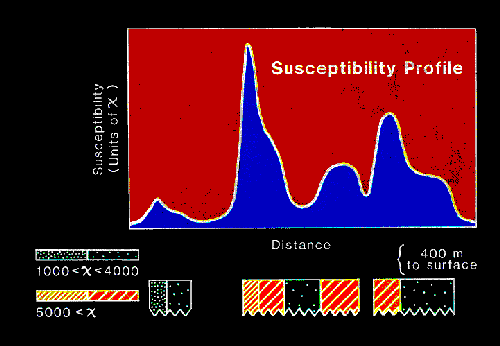Figure 2. Output of a magnetic susceptibility filter.

All of the preceding filters have response increasing exponentially with wavenumber, which makes them sensitive to noise. Applying upward continuation to pre-condition the data does not provide enough smoothing to supress the effect of noise. We undo the smoothing, so to speak, by applying the filter. Reconstruction accuracy depends on retaining only those spectral components of the data which are not corrupted significantly by noise. How much noise is significant depends on how deeply one intends to push the reconstruction. Conditioning too severely stabilizes the filter at the expense of lateral resolution. This results, for example, in the highly smoothed reconstruction of Figure 2. To condition the raw data one might apply Lanczos' (1961) sigma factors over an aperture equal to the expected depth. Another approach is to use measurement reponse smoothing (Hayden, 1987). Measurement response smoothing stabilizes the filter without removing more of its resolving capability than is necessary.

### Werner Deconvolution

ln l953 Werner suggested a method of solving for parameters of a magnetized body that adapts easily to automated processing (Hartmann et al, l972; Jain l975). The method applies generally to gravity, heat-flow, resistivity, and SP data (Kilty, l983). Details are available in any of the above papers, but, briefly stated, Werner deconvolution begins with an assumed model for the field that is a rational function. The coefficients of the rational function relate to the loaction, strength, and polarization of the source. A person can transform the rational function, in turn, into a matrix equation in which the solution vector is a non-linear function of the parameters of the model--that is of the coefficients of the rational function. One goes about solving the matrix equation first, and then calculates the coefficients. Because the system matrix is a function of the observations, Werner deconvolution is non-linear (Tarantola and Valette, 1982).

One advantage of Werner deconvolutlon is that it provides the location of field sources and, thus, frees a person from having to constrain depth with independent information. Another advantage is the simplicity of incorporating interference of neighboring anomalies into the model. The resulting interpretation pays a hefty price for these advantages, though.

Figure 3 illustrates deconvolution of a gravity profile. Every four successive observations provide an estimate of the depth and location of a possible causative body. The crosses or circles each denote a possible solution. Solutions generally follow the basement surface, and one could draw reasonable basement relief from them. There is substantial scatter in the estimates, though, which is the price paid for using the method.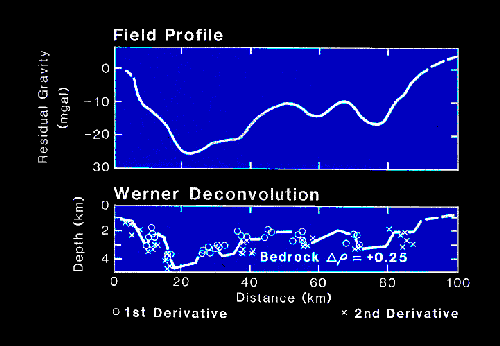Figure 3. A Werner deconvolution.

Some of this scatter arises from aliasing. All implementations of Werner deconvolution resample data at successively greater lntervals to obtain long apertures. Aeromagnetic data is usually so smoothed by its altitude that no significant aliasing occurs in resampling until the aperture is very long. However, ground data is often slightly aliased to begin with. Unless a person smooths these data between successive resamplings, aliasing is bound to occur. Longer apertures then see a particular feature as arising from a successively deeper source. This scatters estimates vertically. Applying an upward continuation filter before resampling adequately eliminates this scatter.

Additional scatter arises from the method itself. Systems of equations characteristic of these methods are ill-conditioned. Condition numbers for the sgstem of Figure 3, for example, vary from 10 to over 8000 depending on interference of neighboring anomalies and the placement of the aperture. Small eigenvalues of such a matrix amplify errors in parameter estimates by large amounts. Less than 1% additive noise in the data easily causes 10% errors in parameter estimates.

Recasting the system in least squares form leads to a better conditioned matrix. lt also provides confidence intervals for the parameters. We have tested a least squares Werner deconvolution on theoretical data and have found the confidence intervals to be disappointingly large. As yet we have not used the least squares algorithm on actual data.

### Exhaustive Search

lP and resistivity data taken ln the dipole-dipole configuration are used to find sulphide and gold deposites. Pseudo-sections, which are the usual method of displaying the data, are difficult to interpret, however, and a method of estimating the location of chargeable sources is needed. Linear filterlng is not possible because sources are not space-invariant in a dipole-dipole survey. Source strength depends on transmitter location. It is possible to form a Werner deconvolution for the problem, but the results are no better than they are for magnetic data. Exhaustive search provides an alternate way of proceeding in this situation.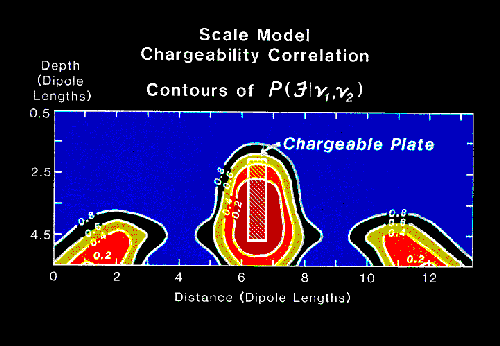Figure 4. An exhaustive search result.

Exhaustive search places a source model in the domain of the problem, does a forward calculation of the resulting field, and compares the calculated results with observations. This continues with every location within the domain of the problem. A convenient means of displaying the results of the calculation is to plot the correlation coefficient, a measure of similarity, between the model and actual data. This lead Robert McEuen to name the method a predictor-correlator. Figure 4 illustrates results of an exhaustine search on a laboratory lP model. The model is a chargeable plate buried in a container of sand. Contours in the figure are of P(F|v1,v2) for the Fisher (F) statistic; found in this case proportional to the ratio of the excess squared deviation of any trial solution to the minumum squared deviation. Note that additional closed contours flanking and below the true plate suggest additional solutions to the problem. The lP data do not admit a unique result.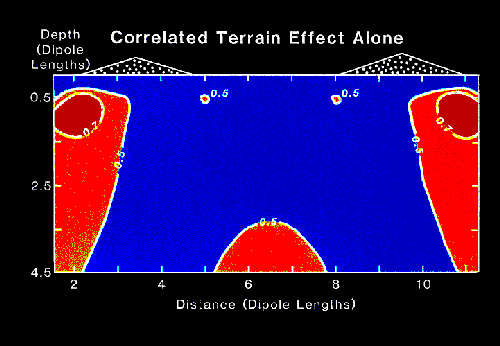Figure 5. Exhaustive search acting on terrain effects alone.

lnstead of using F statistic as a measure, one might use mean squared error or correlation coefficient. For example, Figures 5 and 6 illustrate exhaustive searches of theoretical resistivity pseudo-sections. The model of Figure 5 has uniform resistivity. Terrain effects cause the displayed correlations. ln Figure 6 the same terrain is superimposed on a fault zone having a 1O:1 resistivity contrast with its surroundings. ln the absense of terrain the method would have outlined the fault zone With a 0.9 correlation contour. Compare these results with Figure 7, which shows an objective cross section for actual data using the same analysis model.Figure 6. Exhaustive search acting on terrain and a fault.

The data of Figure 7 come from a discovery breccia near the Promontorio ore body in Sonora, Mexico (West et al, 1983). The contours effectively outline the vertical breccia as drilling delineated it. However, the correlation is not quite as significant as the correlations from terrain effects in figures 5 and 6. Obviously terrain effects would be highly significant in this situation where the correlation with model sources is so slight

.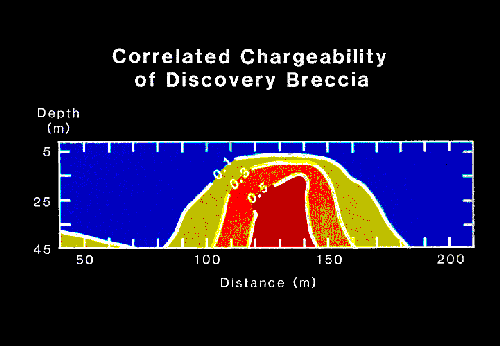Figure 7. Exhaustive search of a breccia discovery zone.

One disadvantage of exhaustive search is that it may be computationally burdensome. The method performs a forward calculation of a model at every point in the domain of the solution for each observed value. ln the general case all this computation cannot be avoided. However, there are methods of making faster calculations in special cases. For example, if the Green's function of the problem is space-invariant and linear the calculations can use a Fourier transform. Consider, for example, a large block of magnetic data which one would examine for a particular target -- a buried drum for instance. One may calculate the field of a drum, call it f, and correlate it wlth the observed data, aall this z, by calculating the inverse to F*Z, where F is the conjugate transform of f and Z is the transform of z. F is a user designed linear filter to calculate correlation.

Figure 8 shows an example. The model is a low-density channel of rectangular cross section. We have specified the depth and dimensions of the channel in detail. The curve in Figure 8 shows correlation of this model against observed gravity data. We calculated correlation with a fast Fourier transform. A horizontal line shows correlation between topography and gravity. Any correlation lying below this line might result from having chosen an incorrect Bouguer reduction density value over part of the profile rather than from the occurrence of a channel. Likely places for a subsurface cavity are wherever the model correlation rises above this line.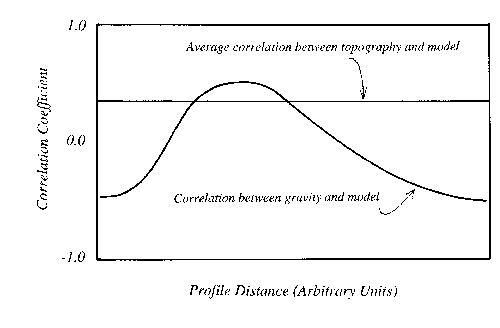Figure 8. Correlation calculated using an FFT.

### lntegrating lndependent Data

One subject we have not covered is how to fold independent data into an objective analyses. One obvious means in exhaustive search is to make the correlation coefficient a linear combination of coefficients calculated separately for each data set. For example, assume we believe that a magnetic anomaly and an IP anomaly arise from the same subsurface body. We can make the correlation coefficient the sum of individual coefficients calculated separately for the lP and magnetic data. Thus, only a high correlation from both data sets provides a high target correlation.

This obvious approach does not work for independent information that can't be calculated from a model. An alternative approach might be to penalize the derived coefficient if the model failed to meet independent constraints. However, we have not thought enough about this problem to say any more at the present time.

### References

• Aronov, V.I., 197O, Optimum filtration of random rrrors in gravimetry. Bull. Soviet Acad. Sci., Earth Physics, no. 10, p. 79-85.
• Hartmann, R.R., Teskey, D.. and Friedberg. J., 1971, A system for rapid digital aeromagnetic interpretation. Geophysics. v. 36. p. 891 -918.
• Hayden, Howard C., l987, Data Smoothing Routine. Computers in Physics. Nov/Dec. p. 74-75.
• Jain, S. 1976, An automatic method of direct interpretation of magnetlc profiles. Geophysics. v. 41. p.531- 541.
• Karous, M., Hjelt, S.E., 1983, Linear filtering of VLP dip-angle measurements. Geophysical Prospecting. v. 31. p. 782-794.
• Karous, M.R., 1979, Effect of relief in EM methods with very distant source. Geoexploration. v. 17, p. 33-42.
• Kilty. K., l983, Werner deconvolution of profile potential field data. Geophysics. v. 48. p. 234-237.
• Lancsoz. C., 1961, Linear Differential Operators. D. Van Nostrand and Company. Princeton, New Jersey.
• Shuey R.T., and P.E. Wannamaker, 1978, Discussion on Application of the generalized inverse to the inversion of static potential data by J. Cribb. Geophysics. v. 43. p. 194-196.
• Tarantola, A. and B. Valette, 1982, Generalized Nonlinear Inverse Problems Using the Least Squares Criterion. Rewiews of Geophysics and Space Physics. v. 2O. p. 2l9-232.
• Werner, S., l953, lnterpretation of magnetic anomalies at sheet-like bodies. Sver. Geol. Undersok. Serv. C, Arsbok 43. no. 6 (cited in Hartmann et al).
• West, R.E., Weiduwilt. W.G., and D.K. Hall, 1983, Discovery of a mineralized breccia pipe using gradient array induced polarizatlon. Geophysics. v. 48., p. 1381-1388.